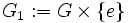# Direct factor not implies characteristic

This article gives the statement and possibly, proof, of a non-implication relation between two subgroup properties. That is, it states that every subgroup satisfying the first subgroup property (i.e., direct factor) need not satisfy the second subgroup property (i.e., characteristic subgroup)
View a complete list of subgroup property non-implications | View a complete list of subgroup property implications
EXPLORE EXAMPLES YOURSELF: View examples of subgroups satisfying property direct factor but not characteristic subgroup|View examples of subgroups satisfying property direct factor and characteristic subgroup

## Statement

A direct factor of a group need not be a characteristic subgroup.

## Proof

### Example of a direct product

Let$G$ be any nontrivial group. Then consider$K = G \times G$, viz., the external direct product of$G$ with itself. The subgroups$G_1 := G \times \{ e \}$ and$G_2 := \{ e \} \times G$ are direct factors of$K$, and are hence both normal in$K$. Note also that they are distinct, since$G$ is nontrivial.

However, the exchange automorphism:$(x,y) \mapsto (y,x)$

exchanges the subgroups$G_1$ and$G_2$. Thus, neither$G_1$ nor$G_2$ is invariant under all the automorphisms, so neither is characteristic. Thus,$G_1$ and$G_2$ are both direct factors of$K$ that are not characteristic.